# Electron Dynamics

## Motion in Combined Electric and Magnetic Fields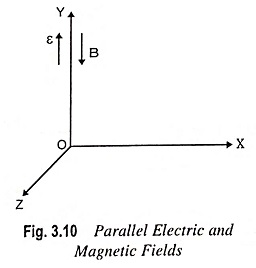Motion in Combined Electric and Magnetic Fields: The Motion in Combined Electric and Magnetic Fields is given by the below two names, namely Parallel Electric and Magnetic Fields Perpendicular Electric and Magnetic Fields Parallel Electric and Magnetic Fields – If both electric and magnetic fields exist simultaneously, the fields being in same or opposite directions, and …

## Motion of an Electron in a Magnetic Field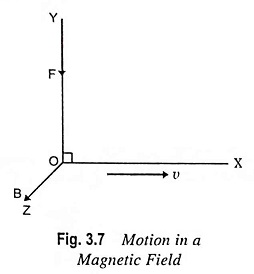Motion of an Electron in a Magnetic Field: Motion of an Electron in a Magnetic Field – Consider an electron to be placed in the region of magnetic field. If an electron is at rest, the force experienced by the particle, Fm = 0 and the particle remains at rest. If an electron moves parallel to the …

## Two Dimensional Motion in a Uniform Electric Field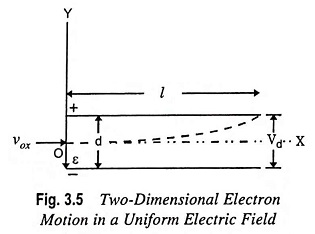Two Dimensional Motion in a Uniform Electric Field: Two Dimensional Motion in a Uniform Electric Field – Let us consider that an electron enters the region between two parallel plates of a parallel plate capacitor oriented as illustrated in Fig. 3.5 with an initial velocity vox m/s in the +X direction. It is assumed that …

## What is Current Density?

What is Current Density? By definition, the current density, denoted by the symbol J, is the current per unit area of the conducting medium i.e., where current I is the rate of flow of charge. If N is the free electron density moving in the conducting medium, e is the charge of electron, then the …

## What is Electron Volt?

What is Electron Volt? The joule (J) is the unit of energy in the SI system. In some engineering power problems this unit is very small, and a factor of 103 or 106 is introduced to convert from watts (1 W = 1 J/s) to kilowatts or megawatts, respectively. For a discussion of the energies …

## Motion of Charged Particle in Electric Field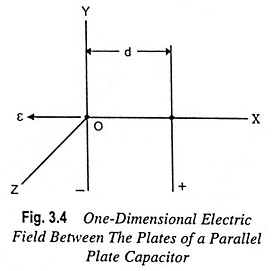Motion of Charged Particle in Electric Field: Motion of Charged Particle in Electric Field – The electric field intensity which is equal to negative of the voltage gradient, is defined as the force (in newtons) acting per unit positive charge (in coulomb), and has the same direction as that of the force. Consequently, the force …

## Rutherford Nuclear Model of Atomic Structure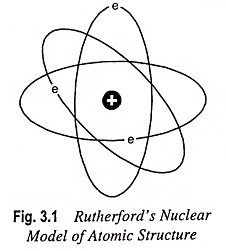Rutherford Nuclear Model of Atomic Structure: In order to explain many phenomena associated with conduction in gases, metals and semiconductors and the electron emission from a metal surface, it is necessary to assume that the atom has loosely bound electrons which can be torn away from it. On the basis of experiments on the scattering …

Scroll to Top